# Thevenin Theorem example quesiton!

#### Chalielogan

Joined Apr 11, 2022
5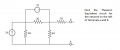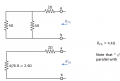Hello. This Thevenin theorem example. However, I know that first step should be turn off all independent sources which is current and voltage source in this circuit. Then find the Rth but the thing is I am confused by the 2 ohm resistor on the left side of terminal a. Why photo next shows that only one 2 ohms resistor to find Rth? Should it be eleminated because 2v voltage source is shorted?

•studyhard24

#### MrAl

Joined Jun 17, 2014
9,155
View attachment 270771View attachment 270772

Hello. This Thevenin theorem example. However, I know that first step should be turn off all independent sources which is current and voltage source in this circuit. Then find the Rth but the thing is I am confused by the 2 ohm resistor on the left side of terminal a. Why photo next shows that only one 2 ohms resistor to find Rth? Should it be eleminated because 2v voltage source is shorted?
Hi,

When you "turn off" or how they state it in several textbooks "kill" a source you have to follow the rule for that source.
For a current source you open circuit it.
For a voltage source you short circuit it.

With this in mind, try again i think it will make more sense.

They probably like to state it as "kill" rather than "turn off" because turning something off can mean different things, but when they say "kill" you have to look deeper into what they actually mean by that and then you find out it is different for different sources. The word "kill" however brings up various connotations of violence and death so maybe better would be "disable" or something like that. So you have to "disable" the sources.

Last edited:

#### RBR1317

Joined Nov 13, 2010
693
They probably like to state it as "kill" rather than "turn off" because turning something off can mean different things,
My textbook (Linear Circuits, Scott) speaks of zeroing the source. A voltage source with zero volts is a short circuit; a current source with zero current is an open circuit.

#### MrAl

Joined Jun 17, 2014
9,155
My textbook (Linear Circuits, Scott) speaks of zeroing the source. A voltage source with zero volts is a short circuit; a current source with zero current is an open circuit.
Hi,

Yeah that sounds good too i guess. Zero means zero of a quantity. It may still be a little confusing though because sometimes it seems like if you disconnect a battery the voltage goes to zero, and it does, except that's not the right way to do it. That would be true of any nomenclature i guess.
I guess we would have to find a word that can describe each way in detail but maybe there is none unless you understand how to apply it too.
"Remove" the source still sounds like you disconnect a battery, which still isnt right.
"Kill" implies you would have to look up how to do each type of source, but can still be confusing if you dont.
"Zero" implies making the source values zero, but doesnt imply that you dont remove the source completely.
"Null Out" might work but they'd still have to look up the meaning of this or take 'null' to mean disconnecting also.
Maybe make up a word that has been never been known anywhere else. Sometimes a foreign language word works for this, but if they know that language i think it fails so maybe a word that does not appear in any language, yet. Here's a somewhat comical example but im sure you could come up with better...
"Gazortize" ha ha, you have to Gazortize the source (big chuckle although something less comical would work better).
That word would force them to look it up and if it was defined it would be found and explain what exactly has to be done.

#### studyhard24

Joined Jun 20, 2022
32
When you determine the Thevenin resistance the current source is considered an open circuit and the voltage source is taken as a short circuit. This is the equivalent resistance for the circuit.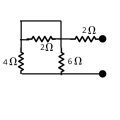Notice that the short circuit line and 2Ohm are in parallel to each other. This 2 Ohm resistor got shorted because there are more paths for the current to pass through than the 2 Ohm.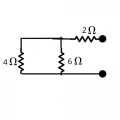Now you can easily understand that the 4 and 6 Ohm Resistors are in parallel. and the equivalent value is in series with the 2 Ohm.

But I'm not sure which technique to apply for determining the Thevenin voltage

Last edited by a moderator:
•Chalielogan

#### Jony130

Joined Feb 17, 2009
5,351
But I'm not sure which technique to apply for determining the Thevenin voltage
For example by changing 5A current source and 4 ohm's resistor into a voltage source in series with 4 ohm's.

•MrAl and studyhard24

#### MrAl

Joined Jun 17, 2014
9,155
Hello again,

Here is another interesting way to solve for the Vth.

We know that if we knew what Vth was since we know what Rth is already, then if we connect a load to the output we would end up with the voltage divider formula to calculate the output voltage:
Vout=(E*RL)/(RT+RL)

and that simply computes what the output voltage is.
Now if we analyze the output using Nodal analysis and keeping RL a variable, we would end up with this:

Vout=(66*RL)/(5*RL+22)

Now if we equate the two we have this:
(E*RL)/(RT+RL)=(66*RL)/(5*RL+22)

and now we only have to get the left into the same form and with the same values as the right side.
An easy way to do this is to make RL=RT because then we know that the output voltage must be 1/2 of E (E/2) and we also get rid of one of the variables already.
Substituting RT in for RL we end up with:
E/2=(66*RT)/(5*RT+22)

and now you should be able to solve this for E.

I like doing it this way because it starts with Nodal Analysis which is a general circuit analysis method that is good to know and keep in practice with. So many problems can be solved with Nodal Analysis that it makes it very well worth knowing.

I would suggest that you try to do this a couple different ways so you should also try this using the method Jony posted just before this reply.

#### Chalielogan

Joined Apr 11, 2022
5
Thank you all for the amazing reply! It helped me a lot!

#### studyhard24

Joined Jun 20, 2022
32
I don't know if you still need to know the process or already done solving this problem. Here is my approach for any newbie struggling to learn. I've already written how to find Thevenin resistance.

For the Voltage,
I've applied the superposition theorem,

Considering the 5Amp only, following the process told by Mr Jony130 converted the circuit into an equivalent voltage source.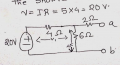After this applying Voltage divider method to find the voltage across 6 Ohm resistance.

v2 = (6/(6+4))*20 = 12 V

Now considering the 2V active only,

the current source becomes open.

This one is way easier. in order to find the voltage across 6 Ohm, simply apply voltage divider rule here also. 4 and 6 Ohm are in series with each other. And it's in parallel with 2 Ohm and the voltage source.

So the voltage across 6 Ohm and 4 Ohm is 2 V.
apply voltage divider rule: v'2 = (6/(6+4))*2 = 1.2V

So the thevein voltage: Vth = v2 + v'2 = 12+1.2=13.2V

#### Attachments

• 1,007.3 KB Views: 1

#### MrAl

Joined Jun 17, 2014
9,155
I don't know if you still need to know the process or already done solving this problem. Here is my approach for any newbie struggling to learn. I've already written how to find Thevenin resistance.

For the Voltage,
I've applied the superposition theorem,

Considering the 5Amp only, following the process told by Mr Jony130 converted the circuit into an equivalent voltage source.
View attachment 271226

After this applying Voltage divider method to find the voltage across 6 Ohm resistance.

v2 = (6/(6+4))*20 = 12 V

Now considering the 2V active only,

the current source becomes open.

This one is way easier. in order to find the voltage across 6 Ohm, simply apply voltage divider rule here also. 4 and 6 Ohm are in series with each other. And it's in parallel with 2 Ohm and the voltage source.

So the voltage across 6 Ohm and 4 Ohm is 2 V.
apply voltage divider rule: v'2 = (6/(6+4))*2 = 1.2V

So the thevein voltage: Vth = v2 + v'2 = 12+1.2=13.2V
Hi,

We are not supposed to hand out full solutions, but it may not matter at this point i think the original poster of this thread understands a lot more than before now.
I do like using superposition too i think it's a good approach as you can often write equations without much trouble.
BTW i get the same result•studyhard24

#### studyhard24

Joined Jun 20, 2022
32
Hi,

We are not supposed to hand out full solutions, but it may not matter at this point i think the original poster of this thread understands a lot more than before now.
I do like using superpositions too I think it's a good approach as you can often write equations without much trouble.
BTW i get the same resultOK Sir, Thank you so much for informing me, Yes this question is an old one, the person asking must have understood it already. I won't write direct answers anymore.

Last edited:

#### MrAl

Joined Jun 17, 2014
9,155
OK Sir, Thank you so much for informing me, Yes this question is an old one, the person asking must have understood it already. I won't write direct answers anymore.
Hi,

Oh that's nice of you thanks. In this case yeah it didnt matter too much and in other cases when the thread gets older or the original thread starter does not show up for a long time we often give complete solutions so any future readers might benefit.